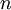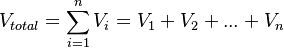# Amagat equation of state

For$n$ components,$V_{total} = \sum_{i=1}^n V_i = V_1 + V_2 + ... + V_n$